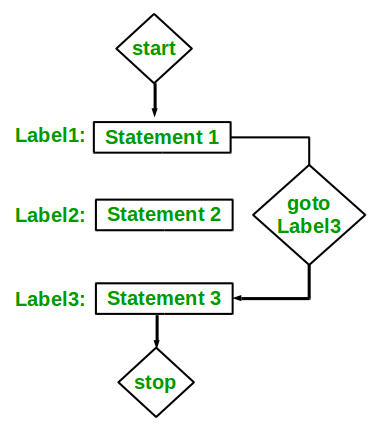# Sum of array Elements without using loops and recursion

Given an array of N elements, the task is to find the Sum of N elements without using loops(for, while & doWhile) and recursion.

Examples:

```Input: arr[]={1, 2, 3, 4, 5}
Output: 15

Input: arr[]={10, 20, 30}
Output: 60
```

## Recommended: Please try your approach on {IDE} first, before moving on to the solution.

Approach: Unconditional Jump Statements can be used to solve this problem.

Unconditional Jump Statements:

Jump statements interrupt the sequential execution of statements, so that execution continues at a different point in the program. A jump destroys automatic variables if the jump destination is outside their scope. There are four statements that cause unconditional jumps in C: break, continue, goto, and return.

To solve this particular problem, goto statement can be useful.

goto Statement:
The goto statement is a jump statement which is sometimes also referred to as unconditional jump statement. The goto statement can be used to jump from anywhere to anywhere within a function.
Syntax:

```Syntax1      |   Syntax2
----------------------------
goto label;  |    label:
.                  |    .
.                  |    .
.                  |    .
label:          |    goto label;
```

In the above syntax, the first line tells the compiler to go to or jump to the statement marked as a label. Here label is a user-defined identifier which indicates the target statement. The statement immediately followed after ‘label:’ is the destination statement. The ‘label:’ can also appear before the ‘goto label;’ statement in the above syntax.Below is the implementation of the above approach:

## C++

 `// C++ program to find the sum of ` `// N elements with goto statement ` ` `  `#include ` `using` `namespace` `std; ` ` `  `// Function to perform desired operation ` `int` `operate(``int` `array[], ``int` `N) ` `{ ` `    ``int` `sum = 0, index = 0; ` ` `  `label: ` `    ``sum += array[index++]; ` ` `  `    ``if` `(index < N) { ` ` `  `        ``// backward jump of goto statement ` `        ``goto` `label; ` `    ``} ` ` `  `    ``// return the sum ` `    ``return` `sum; ` `} ` ` `  `// Driver Code ` `int` `main() ` `{ ` ` `  `    ``// Get N ` `    ``int` `N = 5, sum = 0; ` ` `  `    ``// Input values of an array ` `    ``int` `array[] = { 1, 2, 3, 4, 5 }; ` ` `  `    ``// Find the sum ` `    ``sum = operate(array, N); ` ` `  `    ``// Print the sum ` `    ``cout << sum; ` `} `

## C

 `// C program to find the sum of ` `// N elements with goto statement ` ` `  `#include ` ` `  `// Function to perform desired operation ` `int` `operate(``int` `array[], ``int` `N) ` `{ ` `    ``int` `sum = 0, index = 0; ` ` `  `label: ` `    ``sum += array[index++]; ` ` `  `    ``if` `(index < N) { ` ` `  `        ``// backward jump of goto statement ` `        ``goto` `label; ` `    ``} ` ` `  `    ``// return the sum ` `    ``return` `sum; ` `} ` ` `  `// Driver Code ` `int` `main() ` `{ ` ` `  `    ``// Get N ` `    ``int` `N = 5, sum = 0; ` ` `  `    ``// Input values of an array ` `    ``int` `array[] = { 1, 2, 3, 4, 5 }; ` ` `  `    ``// Find the sum ` `    ``sum = operate(array, N); ` ` `  `    ``// Print the sum ` `    ``printf``(``"%d"``, sum); ` `} `

## C#

 `// C# program to find the sum of ` `// N elements with goto statement ` `using` `System; ` ` `  `class` `GFG ` `{ ` `// Function to perform desired operation ` `static` `int` `operate(``int``[] array, ``int` `N) ` `{ ` `    ``int` `sum = 0, index = 0; ` ` `  `label: ` `    ``sum += array[index++]; ` ` `  `    ``if` `(index < N) ` `    ``{ ` ` `  `        ``// backward jump of goto statement ` `        ``goto` `label; ` `    ``} ` ` `  `    ``// return the sum ` `    ``return` `sum; ` `} ` ` `  `// Driver Code ` `public` `static` `void` `Main() ` `{ ` ` `  `    ``// Get N ` `    ``int` `N = 5, sum = 0; ` ` `  `    ``// Input values of an array ` `    ``int``[] array = { 1, 2, 3, 4, 5 }; ` ` `  `    ``// Find the sum ` `    ``sum = operate(array, N); ` ` `  `    ``// Print the sum ` `    ``Console.Write(sum); ` `} ` `} ` ` `  `// This code is contributed ` `// by Akanksha Rai `

## PHP

 ` `

Output:

```15
```

My Personal Notes arrow_drop_upIf you like GeeksforGeeks and would like to contribute, you can also write an article using contribute.geeksforgeeks.org or mail your article to contribute@geeksforgeeks.org. See your article appearing on the GeeksforGeeks main page and help other Geeks.

Please Improve this article if you find anything incorrect by clicking on the "Improve Article" button below.

Article Tags :
Practice Tags :

5

Please write to us at contribute@geeksforgeeks.org to report any issue with the above content.